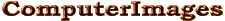Musings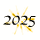# More Excel Magic.Conjure Custom Messages from Excel Text.1

People often use Excel to manage files such as client lists with lots of text, yet many don’t realize they can use formulas to combine and edit these words to produce useful combinations. A few simple formulas and functions are all it takes.

Calculating With Text. Excel calculations are easy. For arithmetic just enter an equal sign followed by the formula. For example the equation =A1+B1 adds numeric values in cells A1 and B1, while =A1*5 multiples the value in A1 by 5. In each case an arithmetic operator (+ - * /) between the entries indicates the desired combination.

Text calculations can combine words in one cell with those in another, for example to join first and last names, and manipulate text entries, such as to pull an initial from a person’s name. The equations look just like arithmetic formulas, but require two special rules for text:

Formula   Result
=A1&B1   JohnDoe
=B1&A1   DoeJohn
=A1&" "&B1   John Doe
=B1&", "&A1   Doe, John

Assumes “John” in A1 and “Doe” in B1.
Formulas Can Combine Text from Different Cells.
• The symbol for com­bining text (text operator) is the amper­sand (&), thus the formula =A1&B1 combines text in cells A1 and B1.
• Text constants go inside quotation marks. For example, =A1&":" adds a colon after the value in A1.

The examples on the right show formulas that combine first and last names from separate cells.

Functions Take Us to the Next Level. Just as with number calculations, functions let us go beyond the basics. Here are a few useful ones:

LEFT. Pulls specified number of characters from the left side of text. Thus if A1 contains “John” the formula =LEFT(A1,1) equals “J”.

RIGHT. Pulls specified number of characters from the right. Thus =RIGHT(A1,5) equals “02108” where A1 contains “Boston, MA 02108”.

Formula   Result
=LEFT(A1,1)&". "&B1   J. Doe
=A1&" "&UPPER(B1)   John DOE

Assumes “John” in A1 and “Doe” in B1.
Functions Can Manipulate Text Entries.

TRIM. Removes all spaces except single spaces between words.

UPPER. Makes text all upper case.

TRIM is useful where lists contain unwanted spaces. For example, some people automatically add a space after a person’s first name because they are used to writing “John Doe” in memos and e-mails. But if the names are in separate cells in Excel, the space shouldn’t be there. The TRIM function can remove it.

Combining Text with Numbers. Sometimes we want to combine text and numbers to create a custom message. For example if A1 contains “apple” and the value in A2 is “5”, the formula

="Thank you for your order of "&A2&" "&A1

produces

Thank you for your order of 5 apples

Formula   Result
=DOLLAR(A1,2)   \$1,000.00
=DOLLAR(A1,0)   \$1,000
=FIXED(A1,2)   1,000.00
=FIXED(A1,0)   1,000
=FIXED(A1,0,TRUE)   1000
=TEXT(A1,"00000")   01000

Assumes value 1000 in A1.
Functions for Formatting Numbers.

Format­ting Num­bers. This works fine until you want to format a number, for example as currency or a date. That requires functions. Three useful functions are:

DOLLAR. Formats number as currency with specified number of decimals.

FIXED. Specifies number of decimal places and whether thousands are separated by commas. “TRUE” as third argument omits commas (see example).

Value   Code   Result
4   mmmm   April
4   mmm   Apr
4   mm   04
4   m   4
5   dddd   Tuesday
5   ddd   Tues
5   dd   05
5   d   5
2016   yyyy   2016
2016   yy   16

Samples for date 4/5/2016.
Custom Date Codes.

TEXT. Formats number using custom format codes. For more about custom codes see Excel Magic.

Putting it all together, with 5000 in cell A1 and 4/5/2016 in B1, the formula:

="Your payment of "&DOLLAR(A1,2)&" is due by "&TEXT(B1,"mmmm d, yyyy")

puts the following message on an invoice:

Your payment of \$5,000.00 is due by April 5, 2016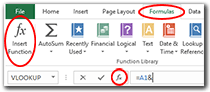Open Function Wizard by Clicking fx Button.

Where Are These Functions? Text functions can be typed directly in the formula bar, but the best way to use them is with the Function Wizard. It shows what functions are available, explains what each does and then helps make sure things are set up correctly.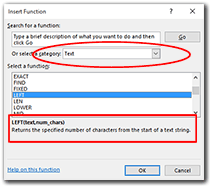First Screen Lists the Functions and What Each Does.

Launch the Function Wizard by clicking the fx button on the left side of the Formula Ribbon (larger button in image) or the smaller button to the left of the Formula Bar (smaller button in image).

The dialogue box that opens has a button for choosing a category to view. Pick “Text” and all Excel’s text functions will be listed. Click on function’s name and its specific syntax and an explanation of what it does will appear at the bottom. Exploring this list is a great way to learn what functions are available and which might be useful.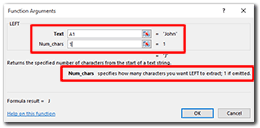Second Screen Shows What Goes Where and Previews Result.

To use a function, click its name in the list and then click OK. A second dialogue box will appear. This window tells you what information you need to provide and where it must go. As you fill in the boxes, the referenced data are shown on the right and the function’s result is previewed. As you go from box to box, an explanation of what each requires appears below. If you need more help, the link at the bottom left corner takes you to the relevant page of Microsoft’s support web site.

Happy conjuring.

-----

1. Billy Rose Theatre Division, The New York Public Library. (1905-20). Brush the mystic: the Hindu basket.